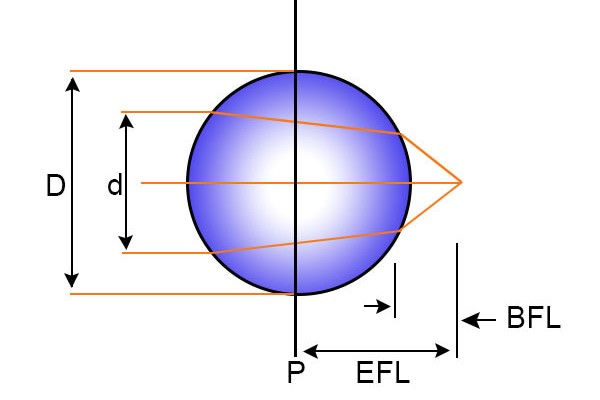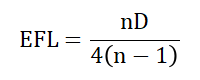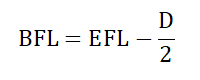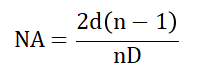# Ball Lens Calculator

This is an online calculator that calculates the Effective Focal Length, Back Focal Length, and Numerical Aperture of a Ball Lens. Just enter the Refractive Index, Diameter of the Ball Lens, and the Diameter of the Input Source to get the corresponding Effective Focal Length, Back Focal Length, and Numerical Aperture value.

• mm
• mm

### Result

• Effective Focal Length
mm
• Back Focal Length
mm
• Numerical Aperture
##### Formula for Ball Lens CalculatorBall lens is a special form of the biconvex lens which is in the shape of a ball (sphere). They are manufactured from a single material, usually solid glass (fused silica) with a diameter of few millimeters or even less than a millimeter. Ball lens is generally used as a collimator and also as a coupling element.The ball lens bends the light falling on it due to refraction. As a result, when rays of light fall on it, they are collimated to a point. Thus they are commonly used as collimators in optical fibers.

There are two focal lengths for a ball lens: effective focal length and back focal length.

Effective focal length (EFL) is the distance between a plane passing through the center of the ball lens and the point where the rays collimate, or the focal point. It is given by,Here D is the diameter of the ball lens and n is the refractive index of the ball lens material.

Back focal length (BFL) is the distance of the focal point to the surface of the ball lens. It is calculated by,For a collimated incident beam, the numerical aperture (NA) of the ball lens depends on the diameter of the ball lens, the refractive index of the ball lens material, and the diameter of the incident beam (input source), d. It is given by,